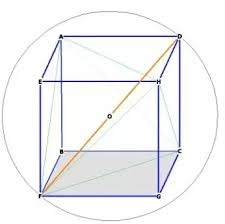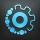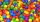# Volume of sphere

How many times does the volume of a sphere increase if its radius increases 2 ×?

n2 =  8

### Step-by-step explanation:Did you find an error or inaccuracy? Feel free to write us. Thank you!Tips to related online calculators
Check out our ratio calculator.
Tip: Our volume units converter will help you with the conversion of volume units.

#### You need to know the following knowledge to solve this word math problem:

We encourage you to watch this tutorial video on this math problem:

## Related math problems and questions:How many times does the surface of a sphere decrease if we reduce its radius twice?
• Cylinder twiceIf the radius of the cylinder increases twice, and the height is reduced twice, then the volume of the cylinder increases (how many times?):
• The EarthThe Earth's surface is 510,000,000 km2. Calculates the radius, equator length, and volume of the Earth, assuming the Earth has the shape of a sphere.
• Ratio of volumesIf the heights of two cylindrical drums are in the ratio 7:8 and their base radii are in the ratio 4:3. What is the ratio of their volumes?
• Area to volumeIf the surface area of a cube is 486, find its volume.
• Sphere VSFind the surface and volume of a sphere that has a radius of 2 dm.
• Cuboid enlargementBy how many percent increases the volume of cuboid if every dimension increases by 30%?
• Cube edgesIf the edge length of the cube increases by 50%, how does the volume of this cube increase?
• Price increaseA 20 percent price increase meant a 90-crown raise. How many cost a product after?
• Sphere A2VThe surface of the sphere is 241 mm2. What is its volume?
• Cube 7Calculate the volume of a cube, whose sum of the lengths of all edges is 276 cm.
• Cube wallsFind the volume and the surface area of the cube if the area of one of its walls is 40 cm2.
• Thousand ballsWe have to create a thousand balls from a sphere with a diameter of 1 m. What will be their radius?
• Rectangular cuboidThe rectangular cuboid has a surface area 5334 cm2, and its dimensions are in the ratio 2:4:5. Find the volume of this rectangular cuboid.
• The potThe pot is a cylinder with a volume of V = 7l and an inner diameter of d = 20cm. Find its depth.
• The prison ballCalculate the density of the material that the prison ball is made from if you know its diameter is 15cm and its weight is approximately 2.3kg. With the help of mathematical-physicochemical tables estimate what material the ball is made from.
• Clay ballsHow many clay balls with a radius of 1 cm can be made from a ball of clay with a radius of 8 cm?